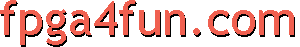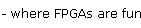## PCI Express 6 - Simple transactions

Let's try to control LEDs from the PCI Express bus.

Xilinx's "Endpoint Block Plus" core allows us to work at the transaction layer level, so it's just going to take us a few lines of code.
Instead of providing data on a 32-bit bus, "Endpoint Block Plus" uses a 64-bit bus (so we get twice as much data at each clock cycle). That's not a problem and a simple state-machine will handle simple memory reads & writes.

```// we use signals from Xilinx's "Endpoint Block Plus"
// first we declare that we are always ready to get data
assign trn_rdst_rdy_n = 1'b0;

// then we create a state machine that triggers when we get a PCI Express memory read or write
reg RXstate;
reg [63:0] RXrd;
always @(posedge clk)
case(RXstate)
// we are going to handle simple memory reads & writes
// we know that with the "Endpoint Block Plus" core, such simple transactions always happens
//  using two cycles so we just need a two-states state machine
// first, we wait for the beginning of a memory transaction with up to 32-bit data (i.e. with length=1)
1'b0: if(~trn_rsrc_rdy_n && ~trn_rsof_n && trn_rd[61:56]==6'b0_00000 && trn_rd[41:32]==10'h001)
begin
RXstate <= 1'b1;
RXrd <= trn_rd;
end
// then the second state waits for the end of the transaction
1'b1: if(~trn_rsrc_rdy_n) RXstate <= 1'b0;
endcase
```

Now we are ready to update the LEDs.

```wire [31:0] RXaddr = trn_rd[63:32];   // memory address (read or write) (valid during the second state of the state machine)
wire [31:0] RXdata = trn_rd[31:0];   // memory data (for a write) (valid during the second state of the state machine)

wire RXrdwr = RXrd;  // 0 for a read, 1 for a write
wire RXRead = ~trn_rsrc_rdy_n & RXstate & ~RXrdwr;	// true when a read is happening
wire RXwrite = ~trn_rsrc_rdy_n & RXstate & RXrdwr;	// true when a write is happening

// update two LEDs using the two LSBs from the data written
reg [1:0] LEDs;
always @(posedge clk) if(RXwrite) LEDs <= RXdata[1:0];
```

For a memory write, that's all there is to it. For a memory read, you need to create a response packet with the data to return. Generating an interrupt is also very easy - just assert a signal called "cfg_interrupt_n".

Want more? Check Dragon-E's startup-kit for a more complete example, and Xilinx's Endpoint Block Plus specification documentation for a description of all the signals.